# The farm

The farm harvested 390 tons of grain. Wheat was 15% more than barley, and rye was 126 tons less than wheat and barley combined. How many tons of wheat, how many tons of barley, and how much rye did the team get?

j =  120
p =  138
r =  132

### Step-by-step explanation:

p = 1.15j
r = p + j - 126
p+j+r = 390

p = 1.15•j
r = p + j - 126
p+j+r = 390

1.15j-p = 0
j+p-r = 126
j+p+r = 390

j = 120
p = 138
r = 132

Our linear equations calculator calculates it.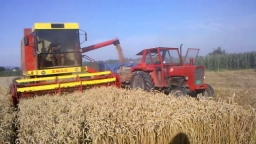Did you find an error or inaccuracy? Feel free to write us. Thank you!Tips to related online calculators
Do you have a system of equations and looking for calculator system of linear equations?

## Related math problems and questions:

• The farmer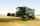The farmer harvested 840 tons of grain in 2006, which was 44% less than in 2005, and one-fifth more than in 2004. How many tons of grain harvested in years 2005 and 2004?
• Freight train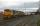The train carries 525 tons of limestone in 29 wagons. Wagons are 15 tonne and 20 tons. How many is 15 ton and how many is 20 ton wagons?
• Bronze, tin and copper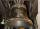Bronze is an alloy of tin and copper. An alloy of 10% tin and 90% copper is Gunmetal. If it contains 20% tin and 80% copper, it is bell metal. How many tons of molten bell metal and how many tons of copper is needed to make 100 tons of Gunmetal?
• RepairingThree employees earned a total of € 469 for repairing the equipment. They split so that the first got 20% more than the second, and the third 15% more than the second. How many euros did everyone get?
• Barrel with liquid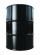Barrel with grain weight 297 kg. When it shed 48% of grain, ha weight 174 kg. What is the weight of empty barrel?
• Geography testsOn three 150-point geography tests, you earned grades of 88%, 94%, and 90%. The final test is worth 250 points. What percent do you need on the final to earn 93% of the total points on all tests?
• Four numbersThe first number is 50% second, the second number is 40% third, the third number is 20% of the fourth. The sum is 396. What are the numbers?
• Three granaries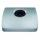Three granaries hold 7,800 metric cent (q) of grain. In the first, 70 tonnes of grain was more than the second and in the third it was 120 tonnes less than in the second. How many grain were in each granary?
• Annual incomeThe annual income (in thousands of \$) of fifteen families is 60, 80, 90, 96, 120, 150, 200, 360, 480, 520, 1060, 1200, 1450, 2500, 7200. Calculate the harmonic and geometric mean.
• Cinema ticketsCinema sold 180 tickets this Thursday, which is 20%. Monday 14%, Tuesday 6%, Wednesday 9%, Friday 24%, Saturday 12%, and Sunday 15%. How many tickets were sold per week?
• Percents from percentHow much is 13% of 20% of 500 greater than 8% of 14% of 200?
• Crowns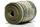1180 crowns are divided into three people: A got 20% less than B and C by 15% more than B. How many got A, B, and C?
• Acid solutionBy adding 250 grams of a 96% sulfuric acid solution to its 3% solution, we changed its initial concentration to 25%. How many grams of 3% of the acid was used for dilution?
• Energy savingThey were released three different, independent inventions saving 20%, 24% and 15% energy. Some considered that while the use of these inventions, the total savings will be 20% + 24% + 15% = 59%. Is this true? How much percent of energy will save all thre
• 15 teachers15 teachers teach for a combined amount of 128 days over a period of 64 days. What is this expressed as a percentage?
• On a farm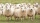On a farm, there are 90 rabbits, 700 sheep, 300 cattle and 500 pigs. What percentage of the total number of animals are rabbits?
• MegapizzaMegapizza will be divided among 100 people. First gets 1%, 2nd 2% of the remainder, 3rd 3% of the remainder, etc. Last 100th 100% of the remainder. Which person got the biggest portion?